# Olympiad Test: Coding Decoding - 1

## 10 Questions MCQ Test Mathematical Olympiad Class 8 | Olympiad Test: Coding Decoding - 1

Description
Attempt Olympiad Test: Coding Decoding - 1 | 10 questions in 20 minutes | Mock test for Class 8 preparation | Free important questions MCQ to study Mathematical Olympiad Class 8 for Class 8 Exam | Download free PDF with solutions
QUESTION: 1

### In a certain code, HAND is written as SZMW, then what will be the code of MILK?

Solution:

In this code, a letter is the nth letter from the beginning of English alphabet then in the code the corresponding letter is the nth letter from the end.
HAND → SZMW
then MILK → NROP

QUESTION: 2

### In a certain code TURN is written as VWTP, then how is WALK written in that code?

Solution: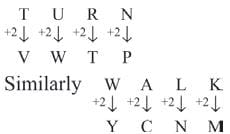QUESTION: 3

### In a coding language GUAVA is coded as HVBWB, then how is JUICE written in that language ?

Solution: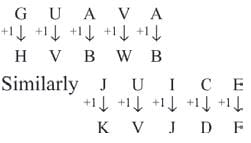QUESTION: 4

In a certain code JUMP is written as ITLO, then how is ROUND written in that code?

Solution: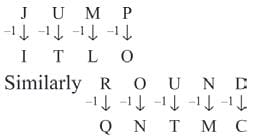QUESTION: 5

If AT = 21; CAT = 24 then what is code for MAT?

Solution:

Position of letters in English alphabet
AT = 1 + 20 = 21.
CAT = 3 + 1 + 20 = 24
MAT = 13 + 1 + 20 = 34

QUESTION: 6

If NAGPUR is written as GPAUNR, Then how STRONG is written in that code?

Solution:

Hence,
NAGPUR → GPAUNR
123456         342516
STRONG → ROTNSG
123456         3425 16

QUESTION: 7

In a certain code CONDEMN is written as CNODMEN. How will TEACHER be written in that code?

Solution: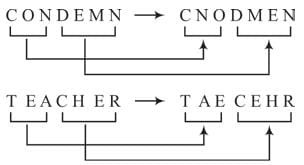In this code, the last letter is as it is in the code. The first letters of the word are divided in two groups of three letters each. In each group the two letters are written in reverse order.

QUESTION: 8

In a coding system, SHEEP is written as GAXXR and BLEAT as HPXTN. How can SEAT be coded?

Solution: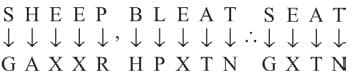QUESTION: 9

If AT = 20; CAT = 60 then what is the value of MAT in that language?

Solution:

AT = 1 × 20 = 20
CAT = 3 × 1 × 20 = 60
MAT = 1 3 × 1 × 20 = 260

QUESTION: 10

If ZIP = 198 and ZAP = 246 then how will you code VIP?

Solution:

In this code,
Z = 2, Y = 3, X = 4, W = 5, ………
N = 14, C = 25, B = 26, A = 27.
ZIP = (Z  + I + P) × 6
= (2 + 19 + 12) × 6 = 198
ZAP = (Z  + A + P) × 6
= (2 + 27 + 12) × 6 = 246
VIP = (V  + I + P) × 6
= (6 + 19 + 12) × 6 = 222.Use Code STAYHOME200 and get INR 200 additional OFF Use Coupon Code